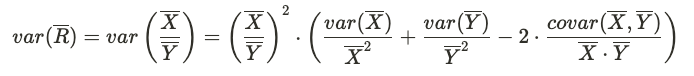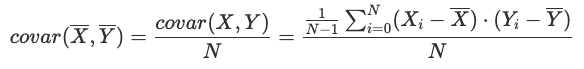# Delta Method

## Delta Method​

Statsig uses the delta method when calculating the variance for variables that have a numerator and denominator.

The variance of ratio and mean metrics depends upon the numerator and denominator variables, which are typically correlated. For example, consider a clicks per session metric. The number of clicks and the number of sessions are two sets of observations coming from the same group of users, so they are not independent from each other. To properly account for this correlation, the variance of the mean of a ratio metric R is obtained using the delta method:where the variance of the numerator and denominator means are computed in the same way as detailed above for count metrics, and the covariance is